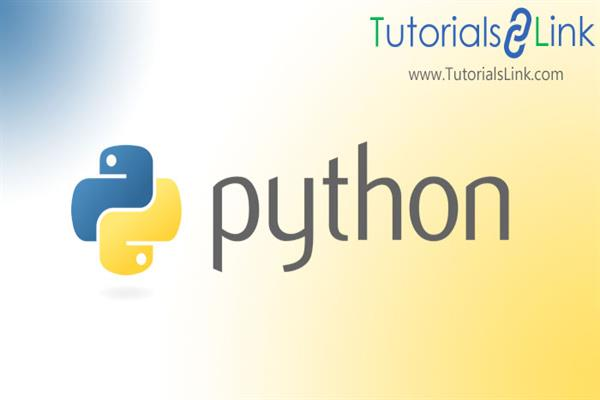Loading, please wait...# What are Python Functions ?

In this article, we will discuss what is python functions, types of functions supported by python, and working of python.

## Introduction:

A function is a set of statements or codes clubbed together to perform a specific task. Functions divide programs into smaller modules. The idea behind developing functions is to create a block of code to perform the repetitive task easily without rewriting the code. It increases reusability, modularity, memory management of programs while decreasing the complexity. Although python offers many built-in functions such as print() etc, one can create their own functions too.

### Function Syntax in Python:

``````function_name(parameters):
Executable statements``````

Here “def” is the keyword which tells the interpreter that the function is being declared here. The keyword is then followed by the name of the function then parameters to be passed to the function are enclosed within parenthesis. Colon (:) indicates the end of the def. After definition, executable statements are written with the indentation in the next line.

### Calling a function:

To call the function, use the name of the function followed by parameters enclosed within the parenthesis.

Syntax: function_name(parameters)

Example:

``````def out():
printf(“In out function”)

out()
``````

In the editor: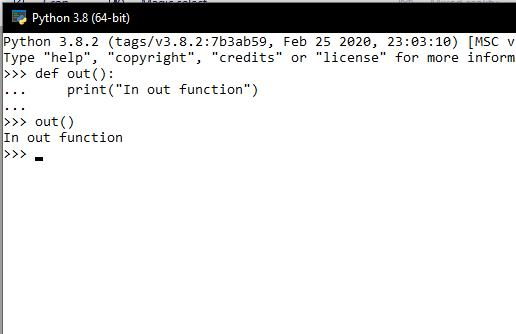Here in this example, we have created simple functions without parameters.

##When a call is made interpreter matches the signature of the calling function with available functions. Here, the signature means the name and parameters of the function. In match is not found, the interpreter will throw an error. If a match is found, the control will be passed on to the function. Function statements will be executed and control will be return back to the caller as soon as control will encounter the “return” statement.

## Types of function: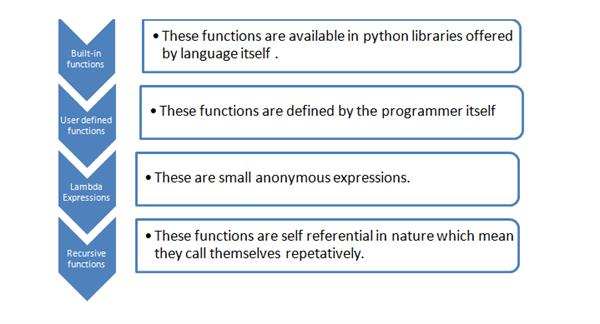### 1. Built-in functions:

Built-in functions are the ones that are offered by the language. These functions are already present in the libraries of the language. The developers only need to call the function to get the work done. Example bool(), abs(), print() etc.

Syntax: name_of_the_function(parameters)

To use any built-in function one must follow the format.

### 2. User-defined functions:

These are the functions that are defined by the user. Here user means the developer of the program. Once the function is created it can be called anywhere within the same program. The scope of the function lies within the program.

### 3. Lambda Expression:

A lambda expression is a small anonymous function. This function can take any number of variables but can have only one expression to evaluate value.

Syntax: variable_name = lambda parameter_1, parameter_2: Expression

Example:

``````x = lambda a,b: a+b
print(x(5,6))``````Then call the function using variable and passing parameters along with it.

### 4. Recursive Function:

Recursive functions are self-referential functions. Recursion is a mathematical concept, in this function repeats itself in order to evaluate the outcome. While writing recursive function one must be careful enough to create the terminating point of the function.

Example:

``````def factorial(a):
if a == 1:
return a
else:
return a*factorial(a-1)

b = factorial(5)
print(b)``````

In the editor: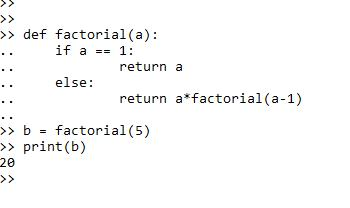Here in this example, we have created a function to evaluate the factorial of a number using recursion.

## Return Statement:

In the above-given example, you might have noticed the “return” keyword. This keyword performs the function of its actual meaning i.e., it returns or goes back from where the function was initially called.

“return” statement gives back the output of the function along with the control back from where it was called. Although the “return” statement is capable of returning many variables. In case the programmer does not return any value, the function will return “none” to the respective variable. When it comes to returning multiple variables, “return” the statement by default use tuples but one can typecast it to return a list of variables rather than a tuple.

Example:

``````def sum(a,b):
x = a+b
return(x)
a = a-1
print(a)

sum(2,4)
``````

In the editor: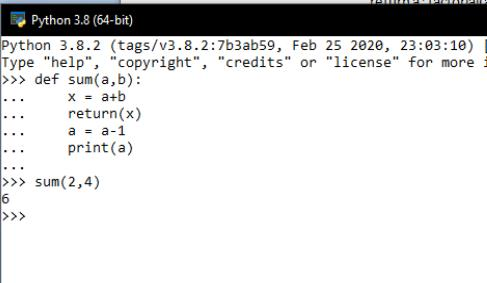“return” statement is used to exit the function. As you can see in the example given above that, code written after the “return” statement is not executed because the return statement sends the control back to the respective variable.

## Pass statement

Till now we have discussed various benefits and ways to use functions but there are some situations in which you have to declare a function but do not want to define it but you cannot leave it blank as well because then the interpreter will give you an error.

Example: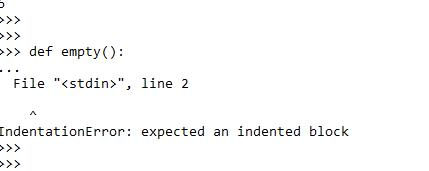So here you can see the error. To remove this error without defining the function, the “pass” statement is used.  In laymen language, the “pass” statement is used when you have to create a function but do not want to write anything in the executable block. For example, assume that you are using a subclass and there is a function you do not want to execute, so you can just simply override the function using the “pass” statement.

Example: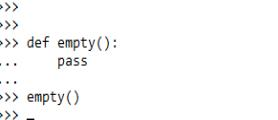After using the pass statement, there is no error. But if there is any code given after the “pass” statement, the code will be executed and the pass statement will be ignored by the interpreter.

Example: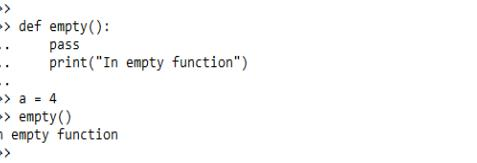So as you know python offers various types of functions in order to ease the programming. Using functions effectively is one trait of a good programmer. So use functions in your program as well and if you have any doubt or query please mention in the comment section below. I will surely solve your query as soon as possible.